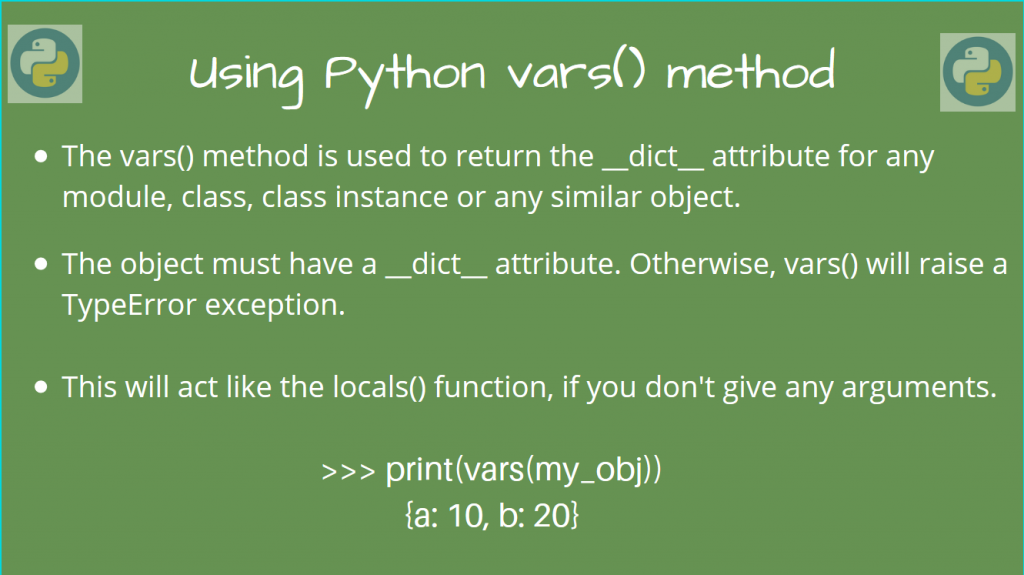# Python vars() – Find the __dict__ attributeHello everyone! In this article, we’ll look at using the Python vars() function, which returns the `__dict__` attribute of an object.

This is rare function, but it helps to see how you can use it, as it is useful in certain situations. Let’s look at these situations now, using illustrative examples!

## Syntax of Python vars()

This function takes an object `obj`, and is of the form:

```vars([obj])
```

This returns the `__dict__` attribute of `obj`, which contains all the attributes of the object which can be writable.

Here, `obj` can be any module / class / instance of a class, etc.

There are a couple of cases here, depending on the argument type and the number of arguments too.

• If you don’t provide any arguments, Python `vars()` will act like the `locals()` method, which returns a dictionary which has the current local symbol table.
• Since it returns the `__dict__` attribute, it the object does not have this attribute, it will raise a `TypeError` exception.

Let’s now look at some examples, related to different objects.

## Using Python vars() without any arguments

As mentioned earlier, this will act as the `locals()` method, and return a dictionary of the local symbol table.

```print(vars())
```

Output

```{'__name__': '__main__', '__doc__': None, '__package__': None, '__loader__': <_frozen_importlib_external.SourceFileLoader object at 0x108508390>, '__spec__': None, '__annotations__': {}, '__builtins__': <module 'builtins' (built-in)>, '__file__': '/Users/vijay/home/python_vars_function.py', '__cached__': None, 'Data': <class '__main__.Data'>, 'd': <__main__.Data object at 0x108565048>, 'math': <module 'math' from '/Library/Frameworks/Python.framework/Versions/3.6/lib/python3.6/lib-dynload/math.cpython-36m-darwin.so'>}
```

## Using Python vars() on a Class Object

If `obj` is a Class Type, or an instance of the class, let’s find out what `vars()` does in this case.

Let’s create an example class `MyClass` and define some attributes on it’s `__init__()` method.

```class MyClass:
def __init__(self, i, j):
self.a = i
self.b = j

m = MyClass(10, 20)
print(vars(m))
print(vars(MyClass))
```

Output

```{'a': 10, 'b': 20}
{'__module__': '__main__', '__init__': <function MyClass.__init__ at 0x000001C24EA129D8>, '__dict__': <attribute '__dict__' of 'MyClass' objects>, '__weakref__': <attribute '__weakref__' of 'MyClass' objects>, '__doc__': None}
```

As you can observe, for the class instance, `vars()` returned all relevant attributes `a` and `b`, along with their values.

Whereas in the case of the class `MyClass`, it is encapsulated under the `main` module, and has the `__init__` method, along with the `__dict__` attribute of the class.

`vars()` calls all of the dunder methods like `__repr__`, `__dict__`, etc.

So, it is more convenient if you can call this function directly rather than calling the dunder methods. (Although there is no difference as such)

Similarly, you can use `vars()` on other objects and classes, like `str` and `list`.

```print(vars(str))
print(vars(list))
```

This will show all relevant attributes and instance methods for both classes.

## Using vars() on a module

We can also use this function on a module, to find out all it’s containing methods, along with other relevant information and even docstrings!

For example, if you want to look at the built-in module `antigravity`, (which is an easter egg!) you do import it, and look at `vars(antigravity)`

```import antigravity

print(vars(antigravity))
```

Sample Output (Last few lines)

``` {'__name__': 'antigravity', '__doc__': None, '__package__': '', 'TimeoutError': <class 'TimeoutError'>, 'open': <built-in function open>, 'quit': Use quit() or Ctrl-Z plus Return to exit, 'exit': Use exit() or Ctrl-Z plus Return to exit, 'copyright': Copyright (c) 2001-2018 Python Software Foundation.

Copyright (c) 1995-2001 Corporation for National Research Initiatives.
}
```

If you use `vars()` on an object (like `int`) which does not have the `__dict__` attribute, it will raise a `TypeError`.

```print(vars(12))
```
```Traceback (most recent call last):
File "vars_example.py", line 12, in <module>
print(vars(12))
TypeError: vars() argument must have __dict__ attribute
```

## Conclusion

In this article, we looked at the Python vars() function, which is useful if you want to quickly get the attributes and all representative methods of any Class/Module/object.

## References

• JournalDev article on Python vars() function